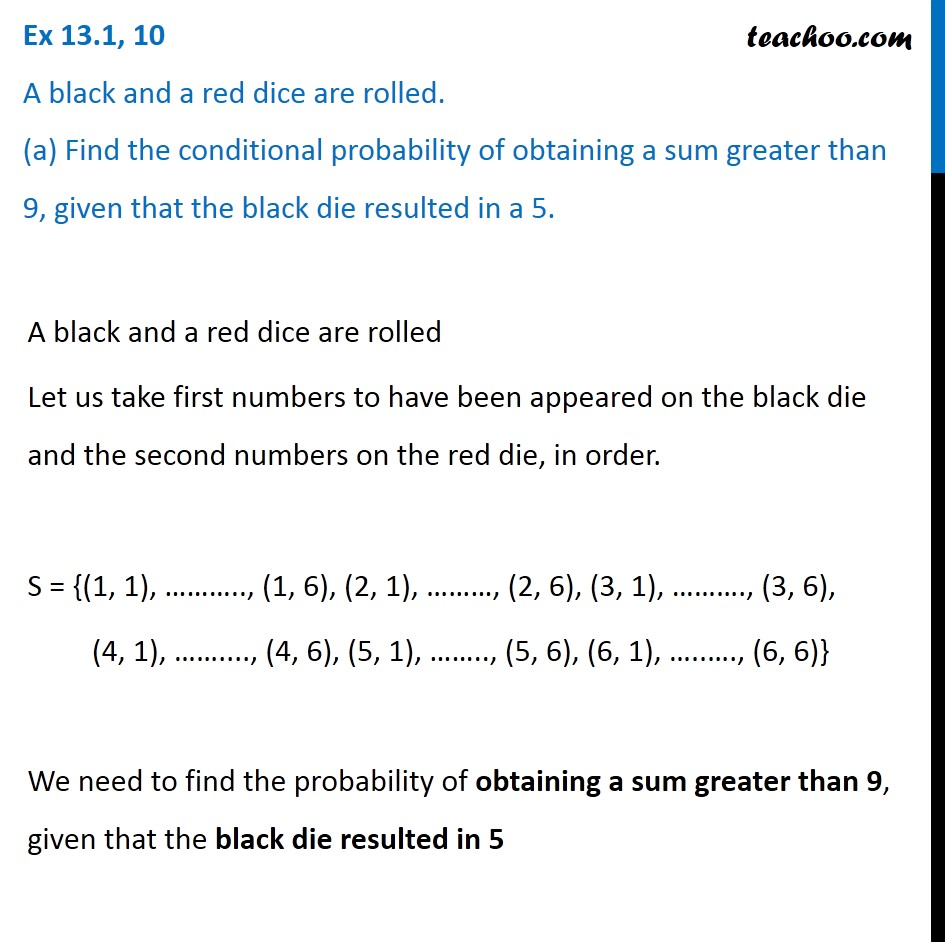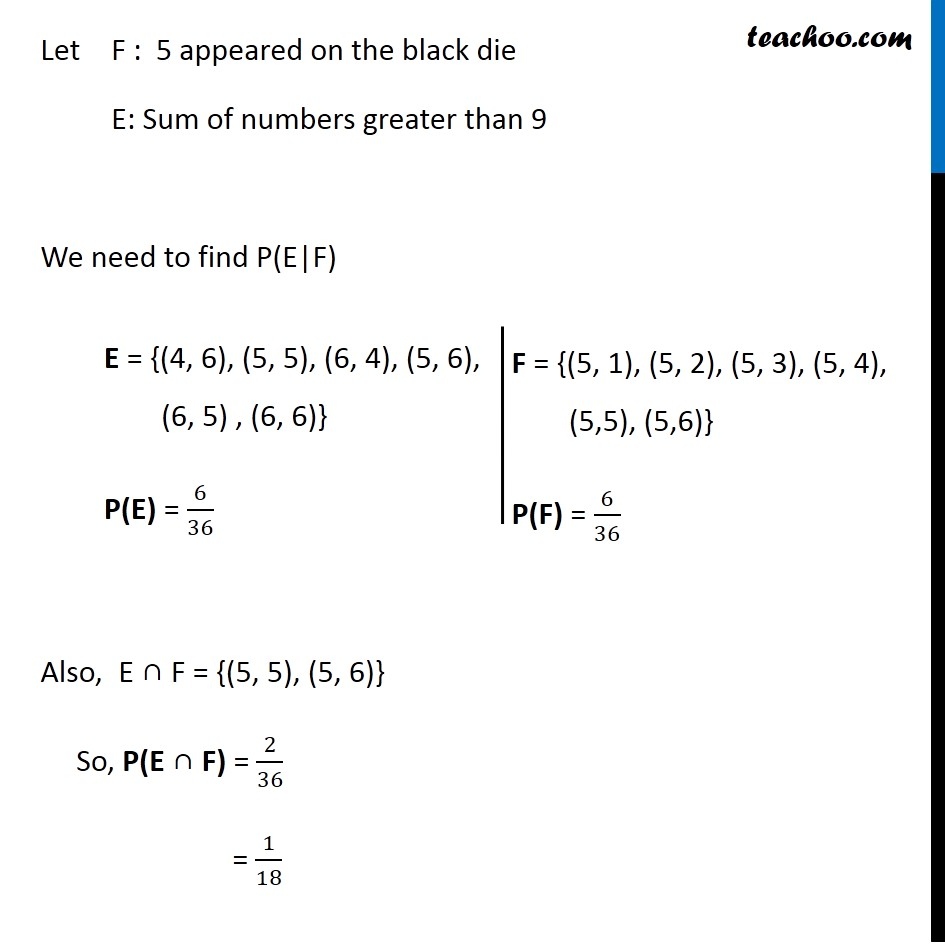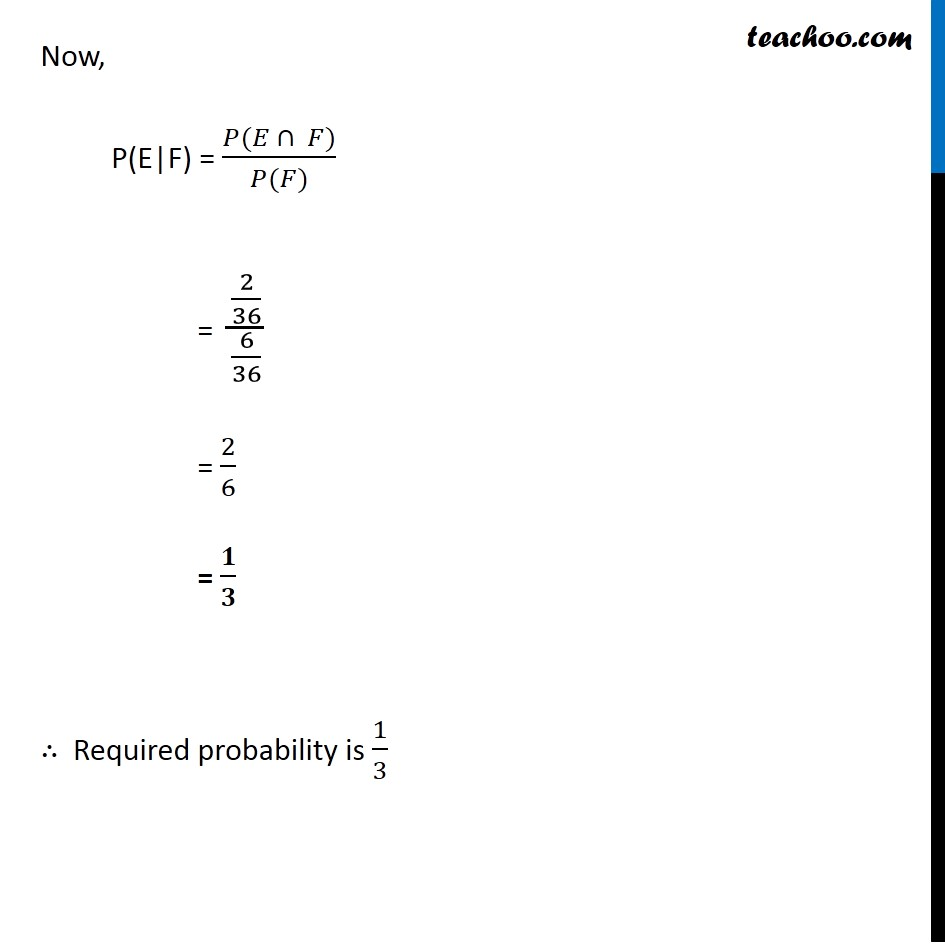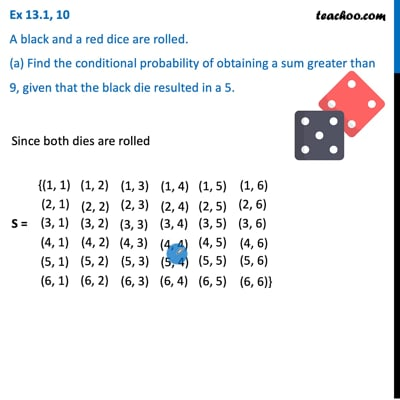Chapter 13 Class 12 Probability

Class 12
Important Questions for exams Class 12This video is only available for Teachoo black users

Learn in your speed, with individual attention - Teachoo Maths 1-on-1 Class

### Transcript

Ex 13.1, 10 A black and a red dice are rolled. (a) Find the conditional probability of obtaining a sum greater than 9, given that the black die resulted in a 5.A black and a red dice are rolled Let us take first numbers to have been appeared on the black die and the second numbers on the red die, in order. S = {(1, 1), ……….., (1, 6), (2, 1), ………, (2, 6), (3, 1), ………., (3, 6), (4, 1), ……...., (4, 6), (5, 1), …….., (5, 6), (6, 1), …..…., (6, 6)} We need to find the probability of obtaining a sum greater than 9, given that the black die resulted in 5 Let F : 5 appeared on the black die E: Sum of numbers greater than 9 We need to find P(E|F) Also, E ∩ F = {(5, 5), (5, 6)} So, P(E ∩ F) = 2/36 = 1/18 E = {(4, 6), (5, 5), (6, 4), (5, 6), (6, 5) , (6, 6)} P(E) = 6/36 F = {(5, 1), (5, 2), (5, 3), (5, 4), (5,5), (5,6)} P(F) = 6/36 Now, P(E|F) = (𝑃(𝐸 ∩ 𝐹))/(𝑃(𝐹)) = (2/36)/(6/36) = 2/6 = 𝟏/𝟑 ∴ Required probability is 1/3 ∴ Required probability is 1/9